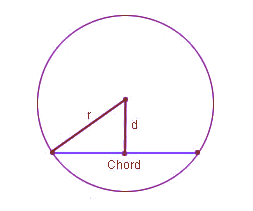# Chord Length Formula

The chord is a line segment that only covers the part inside the circle. A chord that passes through the center of the circle is also a diameter of the circle. There are two basic formulas to find the chord length of the circle.Formula to find the length of a chord of a circle –

$\LARGE Chord\;Length=2r\sin \left (\frac{c}{2}\right )$
$\LARGE Chord\;Length=2\sqrt{r^{2}-d^{2}}$
Where,

r  is the radius of the circle
c  is the angle subtended at the center by the chord
d  is the perpendicular distance from the chord to the circle center
sin is the sine function

## Solved Examples

Question 1: Find the chord of a circle where radius is 7 cm and perpendicular distance from chord to center is 4 cm?

Solution:

Given radius, r = 7 cm

and distance, d = 4 cm

Chord length = $2\sqrt{r^{2}-d^{2}}$

Chord length = $2\sqrt{7^{2}-4^{2}}$

Chord length = $2\sqrt{49-16}$

Chord length = $2\sqrt{33}$

Chord length = 2$\times$5.744

Chord length = 11.48 cm